Geostationary orbitBy Wikipedia,
the free encyclopedia,

http://en.wikipedia.org/wiki/Geostationary_orbit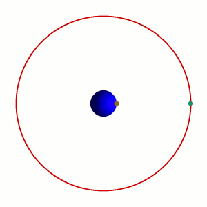Geostationary orbit

A geostationary orbit (GEO) is a geosynchronous orbit directly above the Earth's equator (0° latitude), with a period equal to the Earth's rotational period and an orbital eccentricity of approximately zero. From locations on the surface of the Earth, geostationary objects appear motionless in the sky, making the GEO an orbit of great interest to operators of communications and weather satellites. Due to the constant 0° latitude and circularity of geostationary orbits, satellites in GEO differ in location by longitude only.

The notion of a geosynchronous satellite for communication purposes was first published in 1928 (but not widely so) by Herman Potočnik. The idea of a geostationary orbit was first published on a wide scale in a paper entitled "Extra-Terrestrial Relays — Can Rocket Stations Give Worldwide Radio Coverage?" by Arthur C. Clarke, published in Wireless World magazine in 1945. In this paper, Clarke described it as a useful orbit for communications satellites. As a result this is sometimes referred to as the Clarke Orbit. Similarly, the Clarke Belt is the part of space approximately 36,000 km (22,000 mi) above sea level, in the plane of the equator, where near-geostationary orbits may be implemented. The Clarke Orbit is about 265,000 km (165,000 mi) long.

Geostationary orbits are useful because they cause a satellite to appear stationary with respect to a fixed point on the rotating Earth. As a result, an antenna can point in a fixed direction and maintain a link with the satellite. The satellite orbits in the direction of the Earth's rotation, at an altitude of 35,786 km (22,236 mi) above ground. This altitude is significant because it produces an orbital period equal to the Earth's period of rotation, known as the sidereal day.

## Introduction

Geostationary orbits can be achieved only very close to the ring 35,786 km (22,236 mi) directly high above the equator. This equates to an orbital velocity of 3.07 km/s (1.91 mi/s) or a period of 1436 minutes, which equates to almost exactly one sidereal day or 23.934461223 hours. This makes sense considering that the satellite must be locked to the Earth's rotational period in order to have a stationary footprint on the ground. This can be calculated and verified here. In practice this means that all geostationary satellites have to exist on this ring, which poses problems for satellites that will be decommissioned at the end of their service lives (e.g. when they run out of thruster fuel). Such satellites will either continue to be used in inclined orbits (where the orbital track appears to follow a figure-of-eight loop centered on the equator), or else be elevated to a "graveyard" disposal orbit.

Technically, a "geostationary" orbit is the special case of a geosynchronous orbit which is circular and in the equatorial plane. In practice, however, the terms geosynchronous and geostationary are mostly used interchangeably. Some people in the industry dislike the term "geostationary," because the orbit is not actually stationary (in fact, the term stationary orbit would be an oxymoron), and prefer to use "geosynchronous" because it emphasizes the key point that the orbit is not actually stationary, but synchronized with the motion of the Earth.

A geostationary transfer orbit is used to move a satellite from low Earth orbit (LEO) into a geostationary orbit.

A worldwide network of operational geostationary meteorological satellites is used to provide visible and infrared images of Earth's surface and atmosphere. These satellite systems include:

Most commercial communications satellites, broadcast satellites and SBAS satellites operate in geostationary orbits. (Russian television satellites have used elliptical Molniya and Tundra orbits due to the high latitudes of the receiving audience.) The first satellite placed into a geostationary orbit was the Syncom-3, launched by a Delta-D rocket in 1964.

A statite, a hypothetical satellite that uses a solar sail to modify its orbit, could theoretically hold itself in a "geostationary" orbit with different altitude and/or inclination from the "traditional" equatorial geostationary orbit.

## Derivation of geostationary altitude

In any circular orbit, the centripetal acceleration required to maintain the orbit is provided by the gravitational force on the satellite. To calculate the geostationary orbit altitude, one begins with this equivalence, and uses the fact that the orbital period is one sidereal day.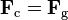$\mathbf{F}_\text{c} = \mathbf{F}_\text{g}$

By Newton's second law of motion, we can replace the forces F with the mass m of the object multiplied by the acceleration felt by the object due to that force: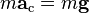$m \mathbf{a}_\text{c} = m \mathbf{g}$

We note that the mass of the satellite m appears on both sides — geostationary orbit is independent of the mass of the satellite. So calculating the altitude simplifies into calculating the point where the magnitudes of the centripetal acceleration required for orbital motion and the gravitational acceleration provided by Earth's gravity are equal.

The centripetal acceleration's magnitude is: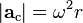$|\mathbf{a}_\text{c}| = \omega^2 r$

where ω is the angular speed, and r is the orbital radius as measured from the Earth's center of mass.

The magnitude of the gravitational acceleration is: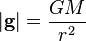$|\mathbf{g}| = \frac{G M}{r^2}$

where M is the mass of Earth, 5.9736 × 10 kg, and G is the gravitational constant, 6.67428 ± 0.00067 × 10 m kg s.

Equating the two accelerations gives: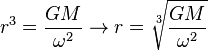$r^3 = \frac{G M}{\omega^2} \to r = \sqrt{\frac{G M}{\omega^2}}$

The product GM is known with much greater accuracy than either factor; it is known as the geocentric gravitational constant μ = 398,600.4418 ± 0.0008 km s: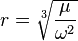$r = \sqrt{\frac\mu{\omega^2}}$

The angular speed ω is found by dividing the angle travelled in one revolution (360° = 2π rad) by the orbital period (the time it takes to make one full revolution: one sidereal day, or 86,164.09054 seconds). This gives: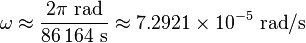$\omega \approx \frac{2 \mathrm\pi~\mathrm{rad}} {86\,164~\mathrm{s}} \approx 7.2921 \times 10^{-5}~\mathrm{rad} / \mathrm{s}$

The resulting orbital radius is 42,164 kilometres (26,199 mi). Subtracting the Earth's equatorial radius, 6,378 kilometres (3,963 mi), gives the altitude of 35,786 kilometres (22,236 mi).

Orbital speed (how fast the satellite is moving through space) is calculated by multiplying the angular speed by the orbital radius: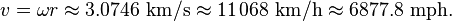$v = \omega r \approx 3.0746~\mathrm{km}/\mathrm{s} \approx 11\,068~\mathrm{km}/\mathrm{h} \approx 6877.8~\mathrm{mph}\text{.}$

## Practical limitations

While a geostationary orbit should hold a satellite in fixed position above the equator, orbital perturbations, such as by the Moon and from the fact that the Earth is not an exact sphere cause slow but steady drift away from the geostationary location. Satellites correct for these effects with station-keeping maneuvers. In the absence of servicing missions from the Earth, the consumption of thruster propellant for station-keeping places a limitation on the lifetime of the satellite.

### Communications

Satellites in geostationary orbits are far enough away from Earth that communication latency becomes very high — about a quarter of a second for a one-way trip from a ground based transmitter to a geostationary satellite and then on to a ground based receiver, and close to half a second for round-trip communication between two earth stations.

For example, for ground stations at latitudes of φ=±45° on the same meridian as the satellite, the one-way delay can be computed by using the cosine rule, given the above derived geostationary orbital radius r, the Earth's radius R and the speed of light c, as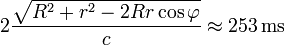$2 \frac {\sqrt{R^2+r^2-2 R r \cos\varphi}} c \approx253\,\mathrm{ms}$

This presents problems for latency-sensitive applications such as voice communication or online gaming.

## Orbit allocation

Satellites in geostationary orbit must all occupy a single ring above the equator. The requirement to space these satellites apart to avoid harmful radio-frequency interference during operations means that there are a limited number of orbital "slots" available, thus only a limited number of satellites can be operated in geostationary orbit. This has led to conflict between different countries wishing access to the same orbital slots (countries at the same longitude but differing latitudes) and radio frequencies. These disputes are addressed through the International Telecommunication Union's allocation mechanism. Countries located at the Earth's equator have also asserted their legal claim to control the use of space above their territory. Since the Clarke Orbit is about 265,000 km (165,000 mi) long, countries and territories in less-populated parts of the world have been allocated slots and reserved portions of the radio-frequency spectrum already, even though they aren't yet using them. The problem presently lies over densely-populated areas such as the Americas and Europe/Africa, and above the middles of the three equatorial oceans.

## Notes and references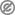This article incorporates public domain material from the General Services Administration document "Federal Standard 1037C" (in support of MIL-STD-188).

Published in July 2009.